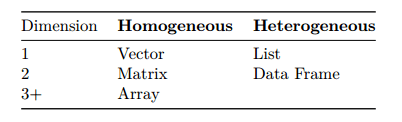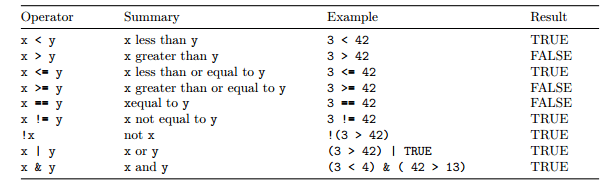### Data and Programming

#### R Objects:

R has five basic or “atomic” classes of objects:

• Numeric – Also known as Double. The default type when dealing with numbers. – Examples: 1, 1.0, 42.5
• Integer – Examples: 1L, 2L, 42L
• Complex – Example: 4 + 2i
• Logical – Two possible values: TRUE and FALSE – You can also use T and F, but this is not recommended. – NA is also considered logical.
• Character – Examples: “a”, “Statistics”, “1 plus 2.”

#### Attributes:

R objects can have attributes, which are like metadata for the object. These metadata can be very useful in that they help to describe the object.

• names, dimnames
• dimensions (e.g. matrices, arrays)
• class (e.g. integer, numeric)
• length
• other user-defined attributes/metadata

#### Data Structures:

R also has a number of basic data structures. A data structure is either homogeneous (all elements are of the same data type) or heterogeneous (elements can be of more than one data type).A list is represented as a vector but can contain objects of different classes.

#### Creating Vectors:

The c() function can be used to create vectors of objects by concatenating things together.

####`x <- c(1,2,3,4,5)x #If you use only x auto-printing occursl <- c(TRUE, FALSE) #logicall <- c(T, F) ## logicalc <- c("a", "b", "c", "d") ## characteri <- 1:20 ## integercm <- c(2+2i, 3+3i) ## complexprint(l)print(c)print(i)print(cm)`

Output:

> print(l)
 TRUE FALSE
> print(c)
 “a” “b” “c” “d”
> print(i)
 1 2 3 4 5 6 7 8 9 10 11 12 13 14 15 16 17 18 19 20
> print(cm)
 2+2i 3+3i

You can also use the vector() function to initialize vectors.

####`x <- vector("numeric", length = 10)length(x) #To get the length of the vector`

Output:

 10

#### Mixing Objects:

Because vectors must contains elements that are all the same type, R will automatically coerce to a single type when attempting to create a vector that combines multiple types.

####`x<- c(100, "Statistics with R", TRUE) #charactery <- c(TRUE, 200) #numericz <- c("a", TRUE) # character`

class(x)
class(y)
class(z)

Output:

> class(x)
 “character”
> class(y)
 “numeric”
> class(z)
 “character”

Remember that the only rule about vectors says this is not allowed. When different objects are mixed in a vector, coercion occurs so that every element in the vector is of the same class.

#### Explicit Coercion:

Objects can be explicitly coerced from one class to another. See the below examples.

####x<- 1:10
class(x)
as.numeric(x)
as.logical(x)
as.character(x)

Output:

> x<- 1:10
> class(x)
 “integer”
> as.numeric(x)
 1 2 3 4 5 6 7 8 9 10
> as.logical(x)
 TRUE TRUE TRUE TRUE TRUE TRUE TRUE TRUE TRUE TRUE
> as.character(x)
 “1” “2” “3” “4” “5” “6” “7” “8” “9” “10”

Sometimes, R also don’t how to coerce an object and this can result in NAs being produced.

####`x <- c("Statistics", "R Programming", "Python")as.numeric(x)as.logical(x)`

Output:

> as.numeric(x)
 NA NA NA
Warning message:
NAs introduced by coercion
> as.logical(x)
 NA NA NA

Frequently you may wish to create a vector based on a sequence of numbers. The quickest and easiest way to do this is with the : operator, which creates a sequence of integers between two specified integers.

####y<-1:10
print(y)

Output:

> print(y)
 1 2 3 4 5 6 7 8 9 10

If we want to create a sequence that isn’t limited to integers and increasing by 2 at a time, we can use the seq() function.

seq(from = 1, to = 10, by = 2)
seq(1.5, 10.2, 2)

Output:

 1.5 3.5 5.5 7.5 9.5

Another common operation to create a vector is rep(), which can repeat a single value a number of times.

####`rep("Statistics", times = 10)x<-c("Statistics","R Programming","Python")rep(x, times = 3)length(x)`

Output:

` "Statistics" "Statistics" "Statistics" "Statistics" "Statistics" "Statistics" "Statistics" "Statistics" "Statistics" "Statistics">> x<-c("Statistics","R Programming","Python")> rep(x, times = 3) "Statistics" "R Programming" "Python" "Statistics" "R Programming" "Python" "Statistics" "R Programming" "Python"> length(x) 3`

####y<- 1:10 #get a sequence from 1 to 10
y[5:10]  #get only last 5 elements
y #get the first element

Output:

 5 6 7 8 9 10
> y #get the first element
 1

#### Vectorization:

One good thing of R is its use of vectorized operations.

####x<- 10:20
y<- x+2
print(y)
2*x
2^x
sqrt(x)
log(x)

Output:

> print(y)
 12 13 14 15 16 17 18 19 20 21 22
> 2*x
 20 22 24 26 28 30 32 34 36 38 40
> 2^x
 1024 2048 4096 8192 16384 32768 65536 131072 262144 524288 1048576
> sqrt(x)
 3.162278 3.316625 3.464102 3.605551 3.741657 3.872983 4.000000 4.123106 4.242641 4.358899 4.472136
> log(x)
 2.302585 2.397895 2.484907 2.564949 2.639057 2.708050 2.772589 2.833213 2.890372 2.944439 2.995732

#### Logical Operators:####x<- 1:10
x<3
x>3
x==3
x == 4 & x != 4
x == 5 | x != 6
x[x > 5]
x[x != 6]
which(x > 5)
x[which(x > 5)]
which(x == max(x))
max(x)
min(x)
range(x)

Output:

> x<- 1:10
> x<3
 TRUE TRUE FALSE FALSE FALSE FALSE FALSE FALSE FALSE FALSE
> x>3
 FALSE FALSE FALSE TRUE TRUE TRUE TRUE TRUE TRUE TRUE
> x==3
 FALSE FALSE TRUE FALSE FALSE FALSE FALSE FALSE FALSE FALSE
> x == 4 & x != 4
 FALSE FALSE FALSE FALSE FALSE FALSE FALSE FALSE FALSE FALSE
> x == 5 | x != 6
 TRUE TRUE TRUE TRUE TRUE FALSE TRUE TRUE TRUE TRUE
> x[x > 5]
 6 7 8 9 10
> x[x != 6]
 1 2 3 4 5 7 8 9 10
> which(x > 5)
 6 7 8 9 10
> x[which(x > 5)]
 6 7 8 9 10
> which(x == max(x))
 10
> max(x)
 10
> min(x)
 1
> range(x)
 1 10

rep() is function sometimes very useful to replicate the values in x.

####rep(1:4, 2)
rep(1:4, each = 2) # not the same.
rep(1:4, c(2,2,2,2)) # same as second.
rep(1:4, c(2,1,2,1))
rep(1:4, each = 2, len = 4) # first 4 only.
rep(1:4, each = 2, len = 10) # 8 integers plus two recycled 1’s.
rep(1:4, each = 2, times = 3) # length 24, 3 complete replications

Output:

> rep(1:4, 2)
 1 2 3 4 1 2 3 4
> rep(1:4, each = 2) # not the same.
 1 1 2 2 3 3 4 4
> rep(1:4, c(2,2,2,2)) # same as second.
 1 1 2 2 3 3 4 4
> rep(1:4, c(2,1,2,1))
 1 1 2 3 3 4
> rep(1:4, each = 2, len = 4) # first 4 only.
 1 1 2 2
> rep(1:4, each = 2, len = 10) # 8 integers plus two recycled 1’s.
 1 1 2 2 3 3 4 4 1 1
> rep(1:4, each = 2, times = 3) # length 24, 3 complete replications
 1 1 2 2 3 3 4 4 1 1 2 2 3 3 4 4 1 1 2 2 3 3 4 4

rev() is a function that  provides a reversed version of its argument.

x<-1:9
rev(x)

Output:

 9 8 7 6 5 4 3 2 1